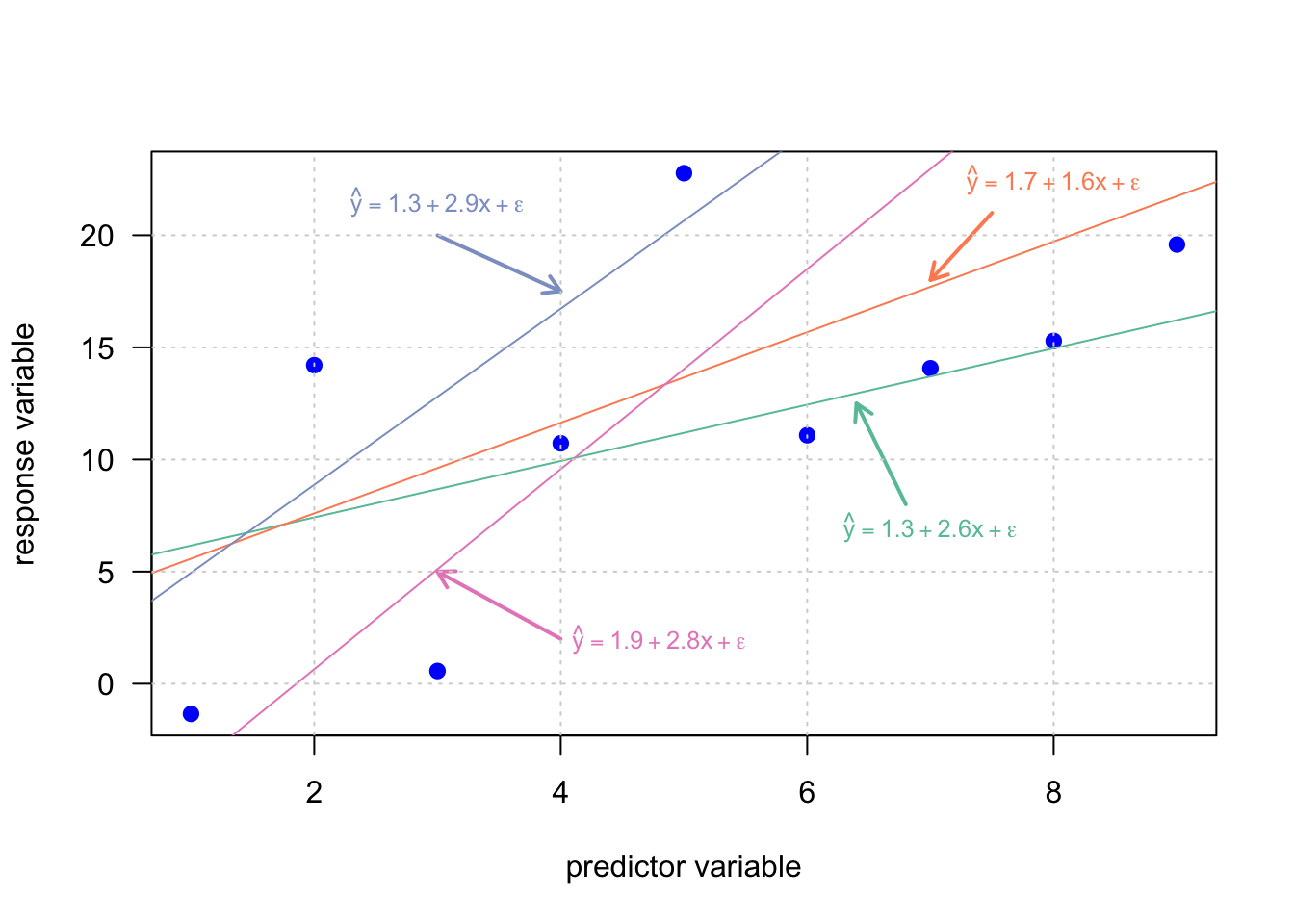Now, as we relaxed the constraints of the deterministic model and introduced an error term $$\epsilon$$, we run into another problem. There are infinitely many regression lines, which fulfill the specifications of the probabilistic model.Obviously, we need a strategy to select the particular regression line, which corresponds to the best model in order to describe the data. In this section we discuss one of the most popular methods to achieve that task, the so called ordinary least squares method (OLS).

As mentioned in the previous section, for each particular pair of values $$(x_1,y_1)$$ the error $$\epsilon_i$$ is calculated by $$y_1-\hat y$$. In order to get the best fitting line for the given data the sum of squares error, denoted by SSE, is minimized:

$min\; SSE = \sum_{i=1}^n \epsilon_i^2=\sum_{i=1}^n (y_i - \hat y_i)^2 \text{.}$

For the simple linear model there exists an analytic solution for $$\beta$$

$\beta = \frac{\sum_{i=1}^n ((x_i- \bar x) (y_i-\bar y))}{\sum_{i=1}^n (x_i-\bar x)^2} = \frac{cov(x,y)}{var(x)}$

and $$\alpha$$:

$\alpha = \bar y - \beta \bar x$

The OLS gives the maximum likelihood estimate for $$\beta$$, when the residuals $$\epsilon$$ are uncorrelated, follow a Gaussian distribution and have equal variance over the entire range of considered predictor variable $$x$$ (homoscedasticity).